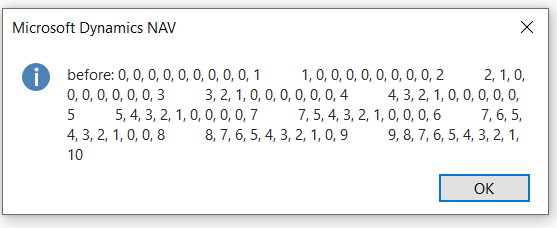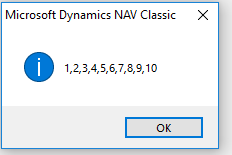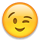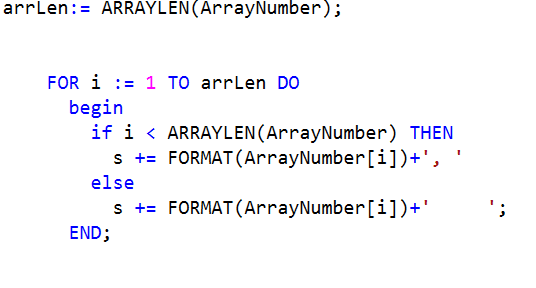#### Howdy, Stranger!

It looks like you're new here. Sign in or register to get started.

# How to output full array by message?

I have an array of 10 elements. How to output this elements by message without hardcoding like:

MESSAGE(FORMAT(ArrayNumber) + ', ' + FORMAT(ArrayNumber) + ', '+ FORMAT(ArrayNumber)
+ ', '+ FORMAT(ArrayNumber) + ', '+ FORMAT(ArrayNumber) + ', '+ FORMAT(ArrayNumber) + ', '
+ FORMAT(ArrayNumber) + ', '+ FORMAT(ArrayNumber)+ ', '+ FORMAT(ArrayNumber)+ ', '+ FORMAT(ArrayNumber))

• You can do this with a loop

i: integer
s: text
```FOR i := 1 TO ARRAYLEN(ArrayNumber) DO
if i<ARRAYLEN(ArrayNumber) THEN
s += FORMAT(ArrayNumber[i]) + ", "
else
s += FORMAT(ArrayNumber[i]);
MESSAGE(s);

```

• You can do this with a loop

i: integer
s: text
```FOR i := 1 TO ARRAYLEN(ArrayNumber) DO
if i<ARRAYLEN(ArrayNumber) THEN
s += FORMAT(ArrayNumber[i]) + ", "
else
s += FORMAT(ArrayNumber[i]);
MESSAGE(s);

```

• Thank you for this answer,

But now I have another problem:

When I input first value it is insert the end of the text variable, so it is OK. But when I want to insert second and third, etc values next situation happening (example on the image).
So how I can produce the variant when all array values are inserting in the text without repeating?• OK fine, but what parameters should get external loop?
• Do you have the loop that builds the text inside another loop?
That's what it looks like to me.
When I use the snipped Phoge posted with an Array I filled with 1-10 it works just fine:Could you post the codesnippets you use for filling the array and for creating the Message?

What does at mean "snipped Phoge"?
• There should be no external loop, was my point.
Or did you just create that message to show the issue with the numbers at the wrong place and the issue isnt multiple outputs of the 10 element array.

If you post the code snippets you are using, I can probably tell you where things go wrong, could be either the array being filled incorrectly, or your code for concatenating the elements into the output string is incorrect.
• What does at mean "snipped Phoge"?
Was a typo, should be "snippet" and Phogue (another typo, damn) is the user that replied to you earlier• There should be no external loop, was my point.
Or did you just create that message to show the issue with the numbers at the wrong place and the issue isnt multiple outputs of the 10 element array.

If you post the code snippets you are using, I can probably tell you where things go wrong, could be either the array being filled incorrectly, or your code for concatenating the elements into the output string is incorrect.

I have this code (the same that Phoge adwised to use)and I insert elements from interface one by one and get the result that I already showed
• Okay, so that part should work just fine, which means the array isn't getting filled correctly.
Can you post the part where you fill the array?
Does the hardcoded solution you posted at the beginning give you the same result as Phogue's solution?

Also again, I'm still not sure if I understand your problem completely, is it:
a) You get the output of several arrays at once. (do you repeat Phogues code without clearing the variable s?)
b) the elements of the array aren't shown in the order you expected.
c) both

Edit: ah sorry missed the part you wrote about inserting. So I guess the code runs multiple times without clearing the text variable s in between. A simple
```CLEAR(s);
```
before the loop should fix that.
• Okay, so that part should work just fine, which means the array isn't getting filled correctly.
Can you post the part where you fill the array?
Does the hardcoded solution you posted at the beginning give you the same result as Phogue's solution?

The hardcoded solution give me right result.
"ArrayNumber" is the variable of array that have dimension 10 . My code work at the next way:
- entering the first array value
- posted the message with this value before sorting and than message that show the result after sorting
- entering second value
- posted the message before sorting and then message after sorting including first and second values
etc.
Maybe problem of repeating is connected with my way of entering the values of the array?
• Hah
I think I know where I have the problem...
• Okay, so that part should work just fine, which means the array isn't getting filled correctly.
Can you post the part where you fill the array?
Does the hardcoded solution you posted at the beginning give you the same result as Phogue's solution?

Also again, I'm still not sure if I understand your problem completely, is it:
a) You get the output of several arrays at once. (do you repeat Phogues code without clearing the variable s?)
b) the elements of the array aren't shown in the order you expected.
c) both

Edit: ah sorry missed the part you wrote about inserting. So I guess the code runs multiple times without clearing the text variable s in between. A simple
```CLEAR(s);
```
before the loop should fix that.

Oh..... Really... I have no explanation how I can missed clear(s)... Thanks a lot)))))))))))) You just have made me happy